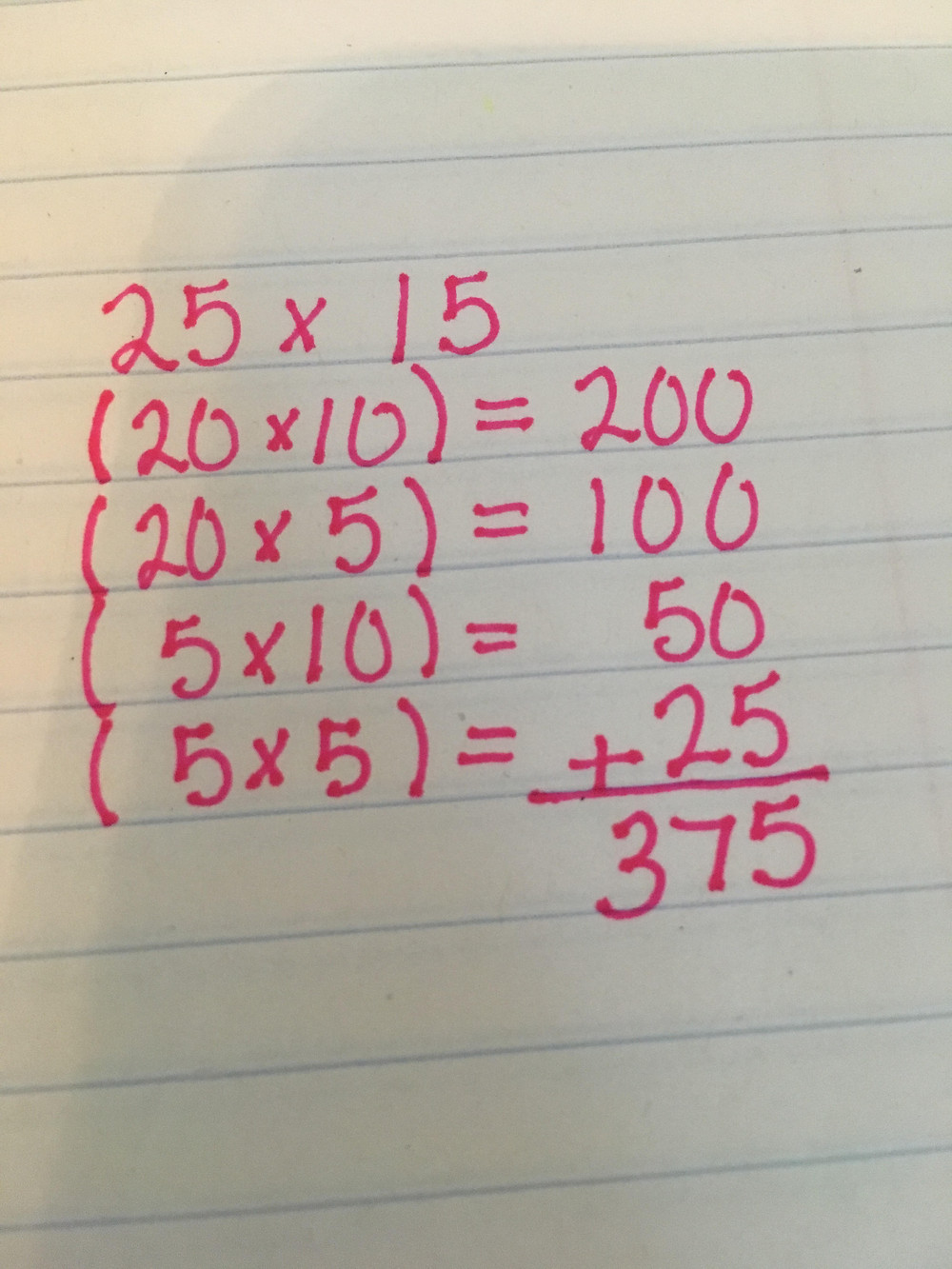My Favorite Multi Digit Multiplication Strategy

Does your child struggle with multi digit multiplication? If so, they are far from alone!

How were you taught how to multiply two digit numbers?

I was taught to multiply two digit numbers using the standard algorithm. My multiplication as an elementary schooler looked something like this:There isn't any problem with this method. It is quick and efficient for those who are proficient with multiplication and understand why the algorithm works the way it does. Deep understanding of why you "carry" the two and add a zero to the second partial product takes quite a bit of exploration and instruction. Since students who are being introduced to multi digit multiplication might not be proficient yet, I always encourage them to explore the numbers and how they can be manipulated while multiplying.

This is where my favorite strategy for multi digit multiplication comes in. If my students are struggling with the standard algorithm, or need more understanding and faster computation I show them how to break down numbers to make the multiplication problem easier. Like this:Or this:When my students start to realize that they can manipulate numbers in this way they understand concepts so much better and often become better at mental math.

Want to know more about how I teach my students this strategy? Check out the video below where I use arrays to help student visualize breaking down multiplication problems.

Does your child need personalized math support this school year? Click here to set up a free online tutoring trial session.

Tags: